# NCERT Solutions for Class 7 Science Chapter 13 – Motion and Time

NCERT Solutions for Class 7 Science Chapter 13 – Motion and Time, contains solutions to various questions in Exercise for Chapter 13.  At the end of the Solutions, all the keywords and Notes which are important to understand Science Chapter 13 Motion and Time Class 7, have been explained in a simple and easy to understand manner. We are providing NCERT Solutions for Class 7 all subjects which can be accessed by clicking here.

## Download NCERT Solutions for Class 7 Science Chapter 13 – Motion and Time

NCERT Solutions for Class 7 Science Chapter 13 – Motion And Time – Solutions to Question 1 to Question 4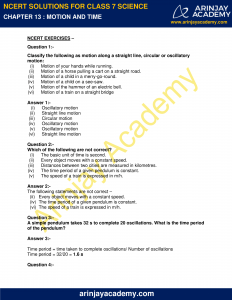NCERT Solutions for Class 7 Science Chapter 13 – Motion And Time – Solutions to Question 4 to Question 6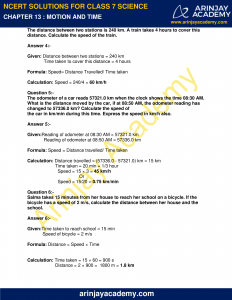NCERT Solutions for Class 7 Science Chapter 13 – Motion And Time – Solutions to Question 7 to Question 9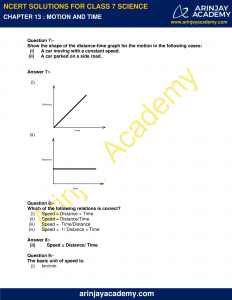NCERT Solutions for Class 7 Science Chapter 13 – Motion And Time – Solutions to Question 9 to Question 11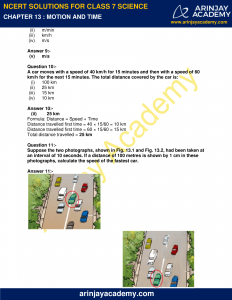NCERT Solutions for Class 7 Science Chapter 13 – Motion And Time – Solutions to Question 11 to Question 13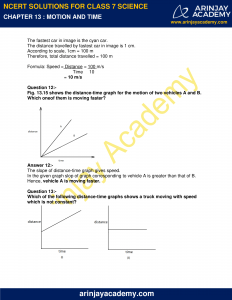NCERT Solutions for Class 7 Science Chapter 13 – Motion And Time – Definition of the Keywords – Bar graph, Graphs, Non-uniform motion, Oscillation, Simple pendulum,Speed, Time period, Uniform motion and Unit of time.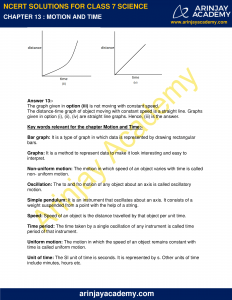NCERT Solutions for Class 7 Science  Chapter 13 – Motion and Time, Exercises includes – Question and Answers which helps you to understand the topic covered in the Motion and Time Class 7 Science, in a better manner to help you to score good marks in your examinations.

### NCERT Solutions for Class 7 Science Chapter 13 – Motion and Time – NCERT Exercises

Question 1:-

Classify the following as motion along a straight line, circular or oscillatory motion:

(i) Motion of your hands while running.

(ii) Motion of a horse pulling a cart on a straight road.

(iii) Motion of a child in a merry-go-round.

(iv) Motion of a child on a see-saw.

(v) Motion of the hammer of an electric bell.

(vi) Motion of a train on a straight bridge

Answer 1:-

(i) Oscillatory motion

(ii) Straight line motion

(iii) Circular motion

(iv) Oscillatory motion

(v) Oscillatory motion

(vi) Straight line motion

Question 2:-

Which of the following are not correct?

(i) The basic unit of time is second.

(ii) Every object moves with a constant speed.

(iii) Distances between two cities are measured in kilometres.

(iv) The time period of a given pendulum is constant.

(v) The speed of a train is expressed in m/h.

Answer 2:-

The following statements are not correct –

(ii)   Every object moves with a constant speed.

(iv)  The time period of a given pendulum is constant.

(vi)  The speed of a train is expressed in m/h.

Question 3:-

A simple pendulum takes 32 s to complete 20 oscillations. What is the time period of the pendulum?

Answer 3:-

Time period = time taken to complete oscillations/ Number of oscillations

Time period = 32/20 = 1.6 s

Question 4:-

The distance between two stations is 240 km. A train takes 4 hours to cover this distance. Calculate the speed of the train.

Answer 4:-

Given: Distance between two stations = 240 km

Time taken to cover this distance = 4 hours

Formula: Speed= Distance Travelled/ Time taken

Calculation: Speed = 240/4 = 60 km/h

Question 5:-

The odometer of a car reads 57321.0 km when the clock shows the time 08:30 AM. What is the distance moved by the car, if at 08:50 AM, the odometer reading has changed to 57336.0 km? Calculate the speed of the car in km/min during this time. Express the speed in km/h also.

Answer 5:-

Given:Reading of odometer at 08:30 AM = 57321.0 km

Reading of odometer at 08:50 AM = 57336.0 km

Formula: Speed = Distance travelled/ Time taken

Calculation: Distance travelled = (57336.0 – 57321.0) km = 15 km

Time taken = 20 min = 1/3 hour

Speed = 15 × 3 = 45 km/h

Or

Speed = 15/20 = 0.75 km/min

Question 6:-

Salma takes 15 minutes from her house to reach her school on a bicycle. If the bicycle has a speed of 2 m/s, calculate the distance between her house and the school.

Answer 6:-

Given:Time taken to reach school = 15 min

Speed of bicycle = 2 m/s

Formula: Distance = Speed × Time

Calculation: Time taken = 15 × 60 = 900 s

Distance = 2 × 900 =  1800 m = 1.8 km

Question 7:-

Show the shape of the distance-time graph for the motion in the following cases:

(i) A car moving with a constant speed.

(ii) A car parked on a side road.

Answer 7:-

(i)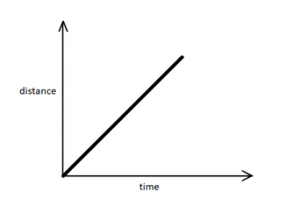(ii)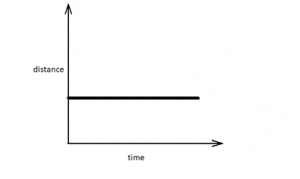Question 8:-

Which of the following relations is correct?

(i) Speed = Distance × Time

(ii) Speed = Distance/Time

(iii) Speed = Time/Distance

(iv) Speed = 1/ Distance × Time

Answer 8:-

(ii) Speed = Distance/ Time

Question 9:-

The basic unit of speed is:

(i) km/min

(ii) m/min

(iii) km/h

(iv) m/s

Answer 9:-

(v) m/s

Question 10:-

A car moves with a speed of 40 km/h for 15 minutes and then with a speed of 60 km/h for the next 15 minutes. The total distance covered by the car is:

(i) 100 km

(ii) 25 km

(iii) 15 km

(iv) 10 km

Answer 10:-

(ii) 25 km

Formula: Distance = Speed × Time

Distance travelled first time = 40 × 15/60 = 10 km

Distance travelled first time = 60 × 15/60 = 15 km

Total distance travelled = 25 km

Question 11:-

Suppose the two photographs, shown in Fig. 13.1 and Fig. 13.2, had been taken at an interval of 10 seconds. If a distance of 100 metres is shown by 1 cm in these photographs, calculate the speed of the fastest car.

Answer 11:-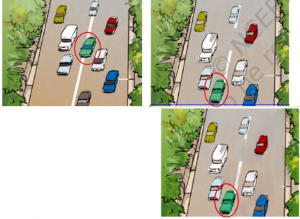The fastest car in image is the cyan car.

The distance travelled by fastest car in image is 1 cm.

According to scale, 1cm = 100 m

Therefore, total distance travelled = 100 m

Formula: Speed = Distance/Time = 100/10 m/s

= 10 m/s

Question 12:-

Fig. 13.15 shows the distance-time graph for the motion of two vehicles A and B. Which oneof them is moving faster?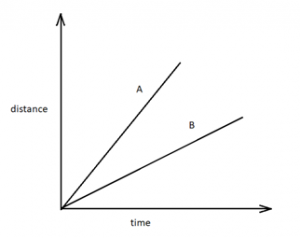Answer 12:-

The slope of distance-time graph gives speed.

In the given graph slop of graph corresponding to vehicle A is greater than that of B. Hence, vehicle A is moving faster.

Question 13:-

Which of the following distance-time graphs shows a truck moving with speed which is not constant?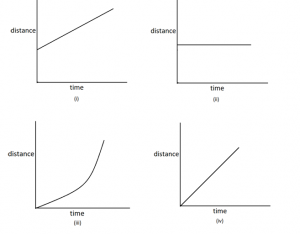Answer 13:-

The graph given in option (iii) is not moving with constant speed.

The distance-time graph of object moving with constant speed is a straight line. Graphs given in option (i), (ii), (iv) are straight line graphs. Hence, (iii) is the answer.

### Topics Covered in Chapter 13 – Motion and Time Class 7 Science :-

• Slow or fast
• Speed
• Measurement of time
• Measuring speed
• Distance time graph

### Important Terms Relevant to understand NCERT Solutions for Class 7 Science Chapter 13 – Motion and Time : –

Bar graph: It is a type of graph in which data is represented by drawing rectangular bars.

Graphs: It is a method to represent data to make it look interesting and easy to interpret.

Non-uniform motion: The motion in which speed of an object varies with time is called non- uniform motion.

Oscillation: The to and fro motion of any object about an axis is called oscillatory motion.

Simple pendulum: It is an instrument that oscillates about an axis. It consists of a weight suspended from a point with the help of a string.

Speed: Speed of an object is the distance travelled by that object per unit time.

Time period: The time taken by a single oscillation of any instrument is called time period of that instrument.

Uniform motion: The motion in which the speed of an object remains constant with time is called uniform motion.

Unit of time: The SI unit of time is seconds. It is represented by s. Other units of time include minutes, hours etc.